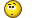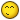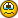d2jsp Forums > Other Games > MMORPG > Guild Wars 1 & 2 > Heroes' Ascent > Full in-depth guide
Group: Member
Posts: 13,034
Joined: Feb 26 2007
Gold: 0.00
Jan 31 2013 08:36pm
Hai you secsy beastsImarobot
Group: Member
Posts: 1,409
Joined: Nov 27 2010
Gold: 0.00
Feb 2 2013 06:58amThis post was edited by Imarobot on Feb 2 2013 06:58am
SharpShot0
Group: Member
Posts: 3,601
Joined: Apr 7 2006
Gold: 0.00
Feb 7 2013 02:45pm
Quote (Motoko @ Dec 28 2012 02:52pm)
I feel like this thread could be included on the history channel.

I can not believe this thread is still up. I haven't HA'd in years and I imagine no one does that still. lol
Motoko
Group: Member
Posts: 3,775
Joined: Nov 27 2008
Gold: 750.00
Feb 14 2013 09:46am
HA WEEKEND WDUPPPPPPPPPPPPPPPPPPPPPPPP^_^ ^_^ ^_^ ^_^ ^_^ ^_^ ^_^ ^_^ ^_^ ^_^ ^_^ ^_^ ^_^ ^_^ ^_^ ^_^ ^_^ ^_^ ^_^ ^_^ ^_^ ^_^ ^_^ ^_^ ^_^ ^_^ ^_^ ^_^ ^_^ ^_^ ^_^ ^_^ ^_^ ^_^ ^_^ ^_^ ^_^ ^_^ ^_^ ^_^ ^_^ ^_^ ^_^ ^_^ ^_^ ^_^ ^_^ ^_^ ^_^ ^_^ ^_^ ^_^ ^_^ ^_^ ^_^ ^_^ ^_^ ^_^ ^_^ ^_^ ^_^ ^_^ ^_^ ^_^ ^_^ ^_^ ^_^ ^_^ ^_^ ^_^ ^_^ ^_^ ^_^ ^_^ ^_^ ^_^ ^_^ ^_^ ^_^ ^_^ ^_^ ^_^ ^_^ ^_^ ^_^ ^_^ ^_^ ^_^ ^_^ ^_^ ^_^ ^_^ ^_^ ^_^ ^_^ ^_^ ^_^ ^_^ ^_^ ^_^ ^_^ ^_^ ^_^ ^_^ ^_^ ^_^ ^_^ ^_^ ^_^ ^_^ ^_^ ^_^ ^_^ ^_^ ^_^ ^_^ ^_^ ^_^ ^_^ ^_^ ^_^ ^_^ ^_^ ^_^ ^_^ ^_^ ^_^ ^_^ ^_^ ^_^ ^_^ ^_^ ^_^ ^_^ ^_^ ^_^
Imarobot
Group: Member
Posts: 1,409
Joined: Nov 27 2010
Gold: 0.00
Feb 25 2013 10:20pmOverdoses
Group: Member
Posts: 7,495
Joined: Aug 14 2006
Gold: 470.00
Mar 30 2013 03:33pm
This isn't where I parked my car.
Motoko
Group: Member
Posts: 3,775
Joined: Nov 27 2008
Gold: 750.00
Apr 8 2013 01:39pm
Quote (Overdoses @ Mar 30 2013 04:33pm)
This isn't where I parked my car.

It would be a great place to park your car.

It would be the only car there and very easy to locate.
Imarobot
Group: Member
Posts: 1,409
Joined: Nov 27 2010
Gold: 0.00
Apr 12 2013 03:55pm
bam
Motoko
Group: Member
Posts: 3,775
Joined: Nov 27 2008
Gold: 750.00
May 10 2013 09:54am
IT IS ALMOST TIME.
kqster
Group: Member
Posts: 1,133
Joined: Jun 9 2010
Gold: 1.00
May 15 2013 07:27am
Quote (Motoko @ May 10 2013 05:54pm)
IT IS ALMOST TIME.

Sooooooo tons of 80 fame wins and holding all night ?

2-3-4k fame over the entire weekend just like the good old days ?

or how active is HA really during these weekends after the release of gw2?
Go Back To Guild Wars 1 & 2 Topic List